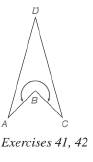Chapter 4.2, Problem 42EElementary Geometry For College St...

7th Edition
Alexander + 2 others
ISBN: 9781337614085

Solutions

Chapter
SectionElementary Geometry For College St...

7th Edition
Alexander + 2 others
ISBN: 9781337614085
Textbook Problem

If the length of side A B ¯ (for kite A B C D ) is 6 in., find the length of A C ¯ (not shown). Recall that m ∠ A = m ∠ C = m ∠ D = 30 ° .To determine

The length of AC¯.

Explanation

Calculation:

Given,

ABCD is a kite

mA=mC=mD=30°

AB=6 in.

Now, draw the line segment AC¯.

In a kite the sum of all the interior angles is 360°.

Thus,

A+B+C+D=360°

30°+B+30°+30°=360°

B+90°=360°

B=360°-90°

B=270°

Therefore, the indicated reflex angle is 270°.

Thus, in ABC B is given by

B=360°-270°

B=90°

Also, in a kite that the pairs of adjacent sides are equal in length

Still sussing out bartleby?

Check out a sample textbook solution.

See a sample solution

The Solution to Your Study Problems

Bartleby provides explanations to thousands of textbook problems written by our experts, many with advanced degrees!

Get Started